JavaScript

# Date Object in Javascript, it’s Syntax and practical use through examples

## Date Object in Javascript:

The date object in Javascript is used to work with date and time. It consists of properties (or constants) and methods. All these properties and methods can be called by directly date object.

The syntax for creating a Date object is:
var dd= new Date()

Where dd represents the variable of type Date. The Date object will automatically hold the current data and time its initial value.

Top Gaming Computers

Best Laptops

Best Graphic Cards

Portable Hard Drives

Best Keyboards

Best High Quality PC Mic

Computer Accessories

## Methods of Date object:

The important and commonly used methods of Date object are as follows:

### Date():

This method returns the current date and time of computer system. The general syntax to use this method is:

Date();

#### The following statement displays the current data & time

```<html>

<script type="text/javaScript">

document.write(Date());

</script>

<body>

</body>
</html>
```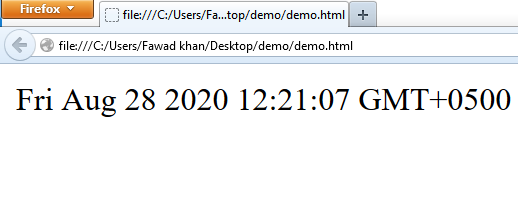### getDate():

This method returns the date of the month. The value returned by this function is between 1 and 31. This method is always used in conjunction with a Date object. The general syntax to use this method is:

dateobject.getDate();

#### The following statements display the day of current month:

```<html>

<script type="text/javaScript">
var d=new Date();
document.write(d.getDate());

</script>

<body>

</body>
</html>
```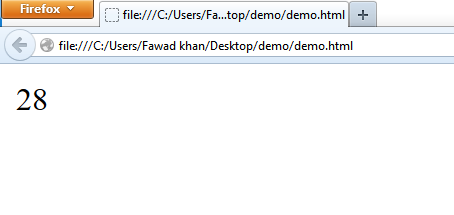getDay():

This method returns the day of week. The value returned by this method is between 0 and 6. Value for Sunday is 0, Monday is 1 and so on. This method is always used in conjunction with a date object. The general syntax to use this method is:

dateObject.getDay();

getMonth():
This method returns the month of a year. The value returned by this function is between 0 and 11. The general syntax to use this method is:

dateobject.getMonth();

getFullYear():

This method returns a four-digit number that represents a year. The general syntax to use this method is:

dateobject.getFullYear();

getHours():

This method returns the hour of a time. The general syntax to use this method is:

dateobject.getHours();

getMinutes():

this method returns the minutes of a time. The general syntax to use this method is:

dateObject.getMinutes();

getSeconds():

This method returns the seconds of a time. The general syntax to use this method is:

dateobject.getSeconds();

setDate():

this method is used to set the day of the month. The general syntax to use this method is:

dateobject.setDate(day);

Where day represents a numeric value (from 1 to 31) of the month. The following statement set the day of month to 10

var d= new Date();

d.setDate(10);

setHours():

This method is used to set the hour of specified time. The general syntax t use this method is:

dataobject.setHours(hour);

where hour represents a numeric value for hour. Its value may be from 0 to 23. The following statement set the hour of current time to 12:

var d= new Date();

d.setHours(12);

setMinutes():

This method is used to set the minutes of specified time.

The general syntax to use this method is:

dateobject.setMinutes(min);

Where min represents a numeric value for minutes. Its value may be from 0 to 59. The following statement set the minutes of current time to 30:

var d= new Date();

d.setMinutes(30);

SetSeconds():

This method is used to set the seconds of specified time.

The general syntax to use this method is:

Dateobject.setSeconds(sec);

Where sec represents a numeric value for seconds. Its value may be from 0 to 59. The following statement set the seconds of current time to 15:

Var d= new Date();

d.setSeconds(15);

setFullYear():

This setFullYear() method is used to set the full year, indicating year, month and day. The general syntax to use this method is

dateobject.setFullYear(year,month,day);

setMonth():

This setMonth method is used to set the month. The general syntax to use this method is:

dateobject.setMonth(month);

setYear():

The setYear() method is used to set the year. The general syntax to use this method is:

dateobject.setYear(year);

## Example: write JavaScript code t display four images randomly in a table cell:

```<html>

<script type="text/javaScript">
images = new Array();
images= "image/test.jpg";
images= "image/test2.jpg";
images= "image/test3.jpg";
images= "image/test4.jpg";
function abc()
{
var imagenumber=4;
var randomnumber=Math.random();
var rand1=Math.round((imagenumber-1)*randomnumber)+1;
var image = images[rand1];
document.getElementById("pic").src=image;
}

</script>

<body>
<script type= "text/javascript">
var x = setInterval("abc()",2000);
</script>

<table border="2">
<tr> <th align= "center">Moving Pictures</th></tr>
<tr>
<td> <img id="pic" src="" border="0" width="180" height="150"></td>
</tr>

</table>
</body>
</html>
```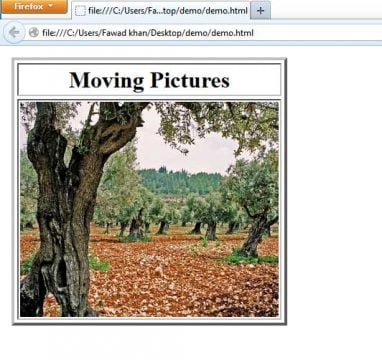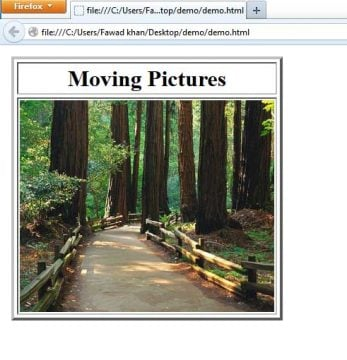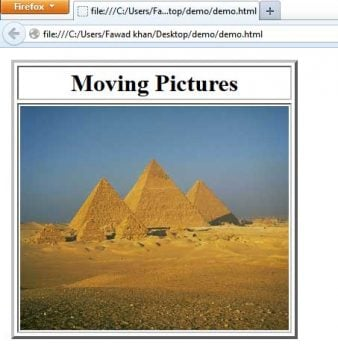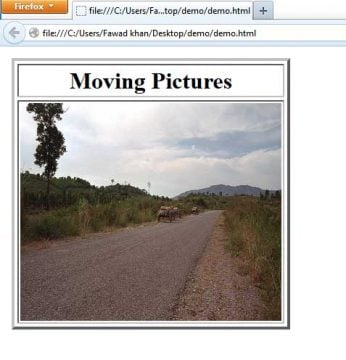## Example: write JavaScript code to create a scrolling message in the browser’s status bar:

```<html>

<script type="text/javaScript">
var msg = "programming digest JavaScript";
var delay = 100;
var startPos= 120;
var pos=0;
function StartScrolling()
{
for(var i =0; i< startPos; i++)
msg=" " + msg;
scroll();
}
function scroll()
{
if (pos< msg.length)
window.status= msg.substring(pos,msg.length);
else
pos=-1;
pos++
setTimeout("scroll()", delay);
}

</script>